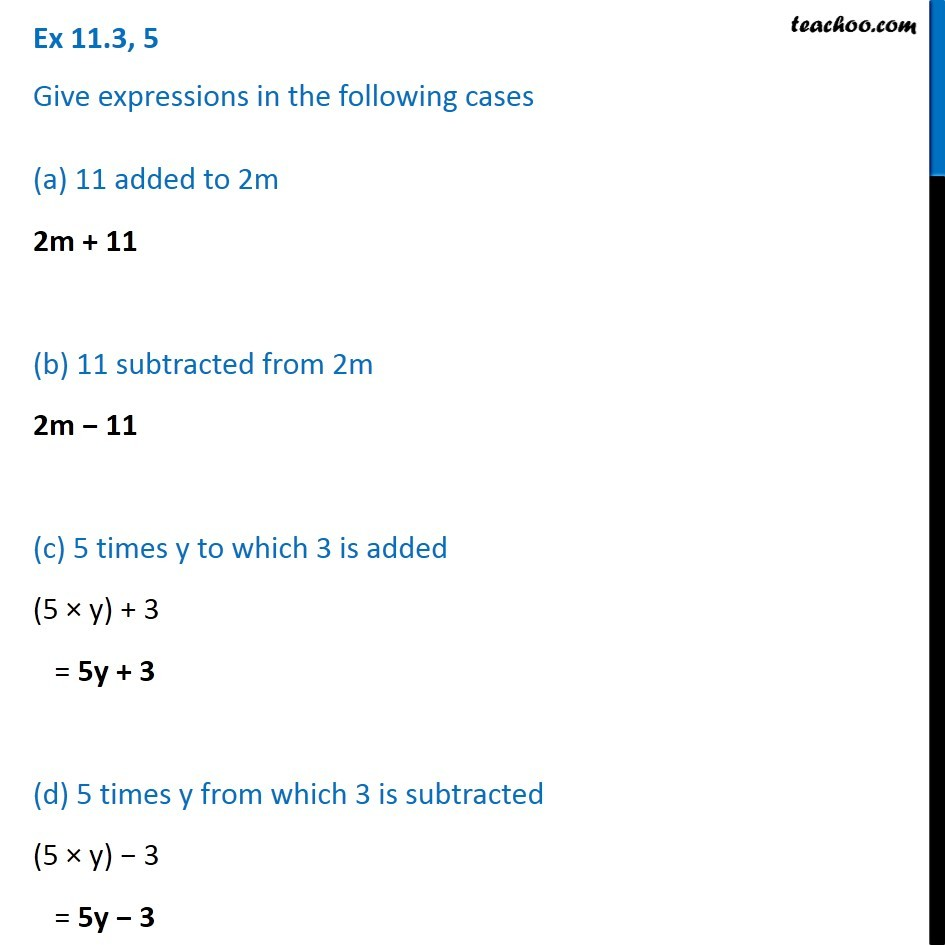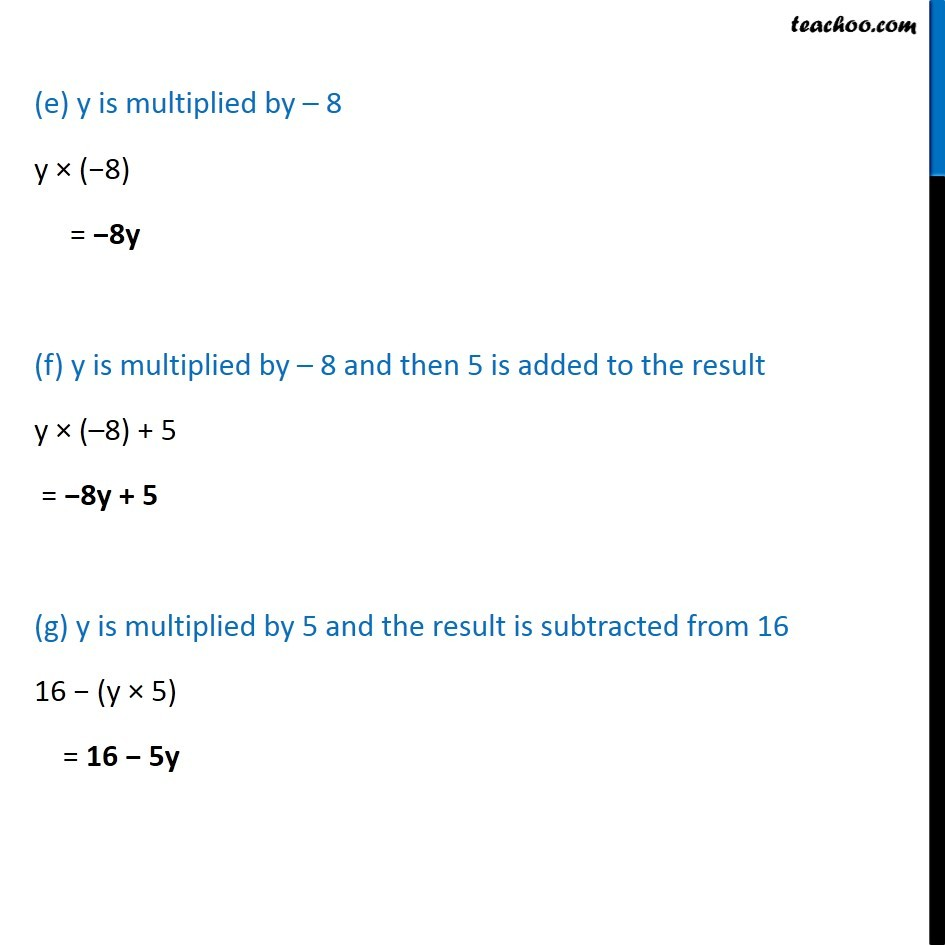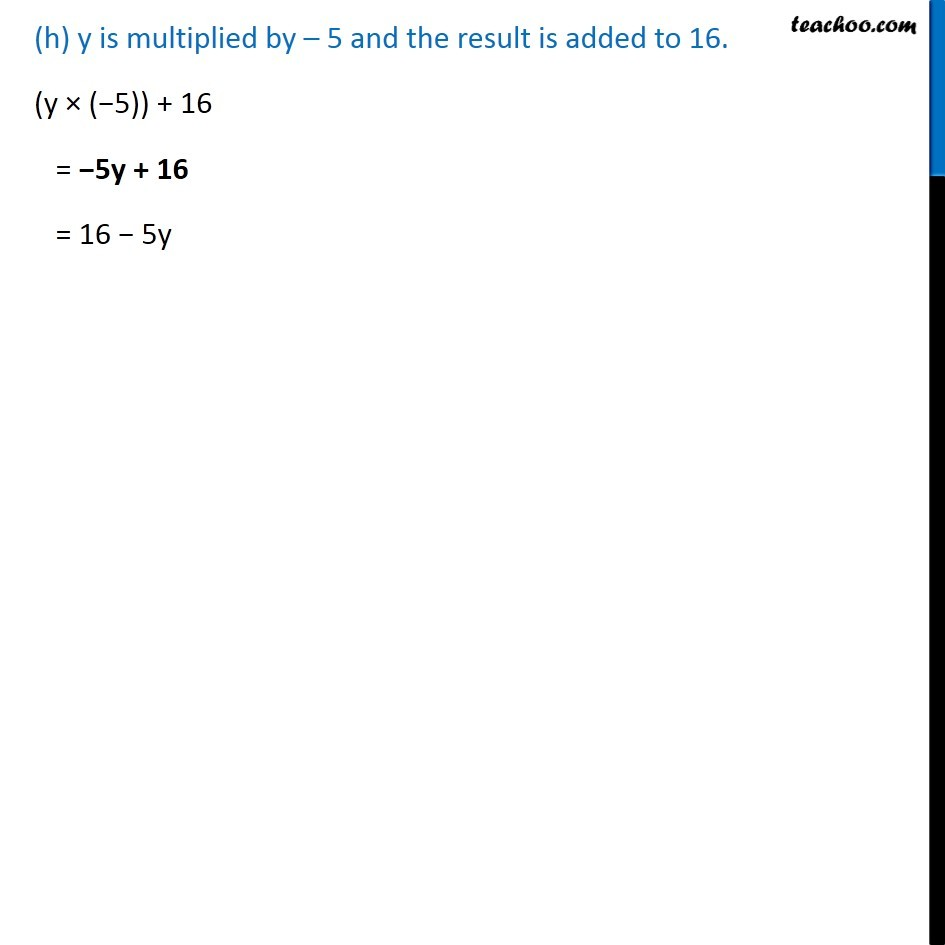Expression with variables

Chapter 11 Class 6 Algebra
Concept wiseIntroducing your new favourite teacher - Teachoo Black, at only ₹83 per month

### Transcript

Ex 11.3, 5 Give expressions in the following cases (a) 11 added to 2m 2m + 11 (b) 11 subtracted from 2m 2m − 11 (c) 5 times y to which 3 is added (5 × y) + 3 = 5y + 3 (d) 5 times y from which 3 is subtracted (5 × y) − 3 = 5y − 3 (e) y is multiplied by – 8 y × (−8) = −8y (f) y is multiplied by – 8 and then 5 is added to the result y × (–8) + 5 = −8y + 5 (g) y is multiplied by 5 and the result is subtracted from 16 16 − (y × 5) = 16 − 5y (h) y is multiplied by – 5 and the result is added to 16. (y × (−5)) + 16 = −5y + 16 = 16 − 5y